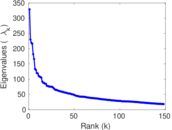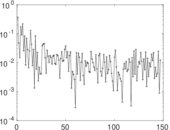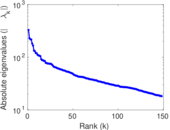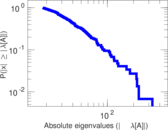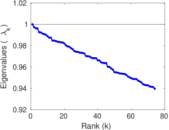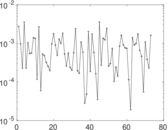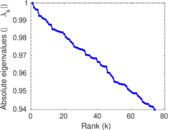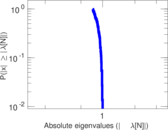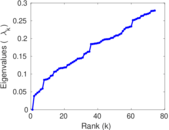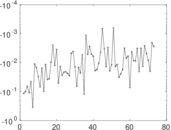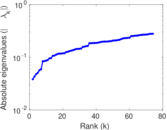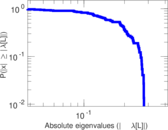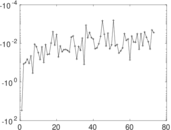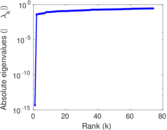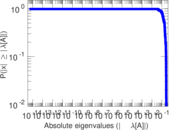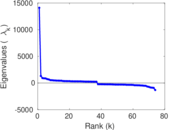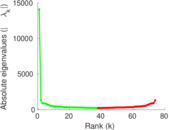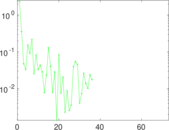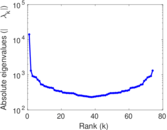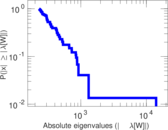# Wikibooks edits (zh)

This is the bipartite edit network of the Chinese Wikibooks. It contains users and pages from the Chinese Wikibooks, connected by edit events. Each edge represents an edit. The dataset includes the timestamp of each edit.

 Code `bzh` Internal name `edit-zhwikibooks` Name Wikibooks edits (zh) Data source http://dumps.wikimedia.org/ AvailabilityDataset is available for download Consistency checkDataset passed all tests Category Authorship network Dataset timestamp 2017-10-20 Node meaning User, article Edge meaning Edit Network formatBipartite, undirected Edge typeUnweighted, multiple edges Temporal dataEdges are annotated with timestamps

## Statistics

 Size n = 14,125 Left size n1 = 2,372 Right size n2 = 11,753 Volume m = 53,881 Unique edge count m̿ = 25,137 Wedge count s = 10,536,148 Claw count z = 6,717,168,528 Cross count x = 3,686,306,958,896 Square count q = 3,482,849 4-Tour count T4 = 70,075,242 Maximum degree dmax = 4,969 Maximum left degree d1max = 4,969 Maximum right degree d2max = 699 Average degree d = 7.629 17 Average left degree d1 = 22.715 4 Average right degree d2 = 4.584 45 Fill p = 0.000 901 675 Average edge multiplicity m̃ = 2.143 49 Size of LCC N = 13,242 Diameter δ = 13 50-Percentile effective diameter δ0.5 = 3.835 57 90-Percentile effective diameter δ0.9 = 5.756 44 Median distance δM = 4 Mean distance δm = 4.521 57 Gini coefficient G = 0.745 403 Balanced inequality ratio P = 0.205 471 Left balanced inequality ratio P1 = 0.121 657 Right balanced inequality ratio P2 = 0.286 056 Relative edge distribution entropy Her = 0.808 014 Power law exponent γ = 2.763 48 Tail power law exponent γt = 2.211 00 Tail power law exponent with p γ3 = 2.211 00 p-value p = 0.012 000 0 Left tail power law exponent with p γ3,1 = 1.821 00 Left p-value p1 = 0.333 000 Right tail power law exponent with p γ3,2 = 2.981 00 Right p-value p2 = 0.000 00 Degree assortativity ρ = −0.207 354 Degree assortativity p-value pρ = 3.311 08 × 10−242 Spectral norm α = 329.194 Algebraic connectivity a = 0.038 271 9 Spectral separation |λ1[A] / λ2[A]| = 1.430 61 Controllability C = 10,398 Relative controllability Cr = 0.746 929

## Plots

### Fruchterman–Reingold graph drawing### Degree distribution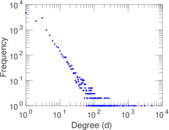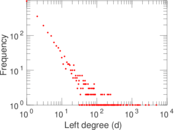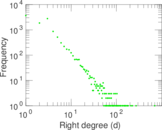### Cumulative degree distribution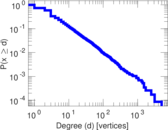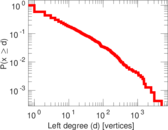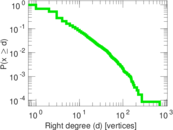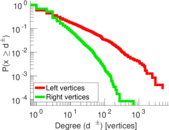### Lorenz curve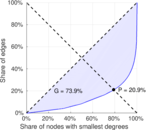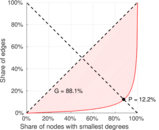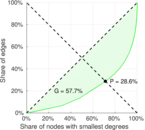### Spectral distribution of the adjacency matrix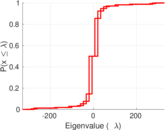### Spectral distribution of the normalized adjacency matrix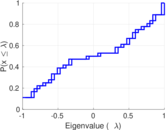### Spectral distribution of the Laplacian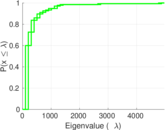### Spectral graph drawing based on the adjacency matrix### Spectral graph drawing based on the Laplacian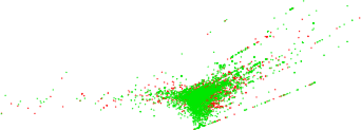### Spectral graph drawing based on the normalized adjacency matrix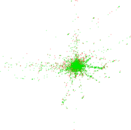### Degree assortativity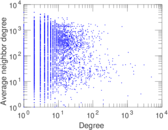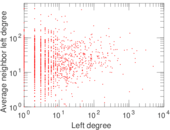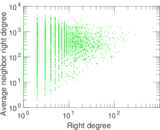### Zipf plot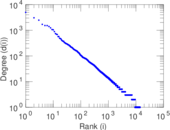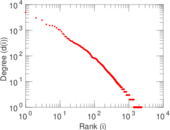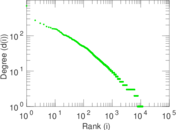### Hop distribution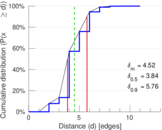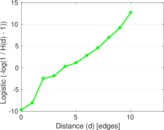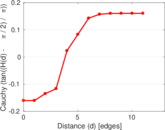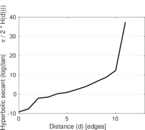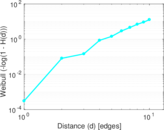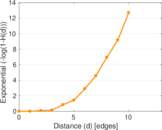### Double Laplacian graph drawing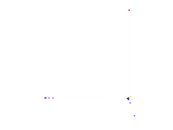### Delaunay graph drawing### Edge weight/multiplicity distribution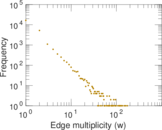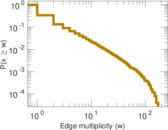### Temporal distribution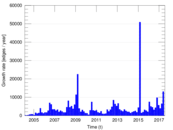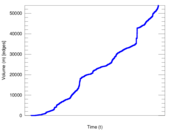### Temporal hop distribution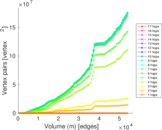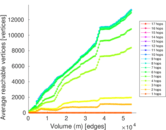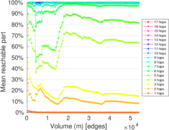### Diameter/density evolution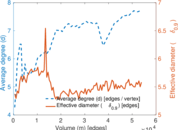### Matrix decompositions plots